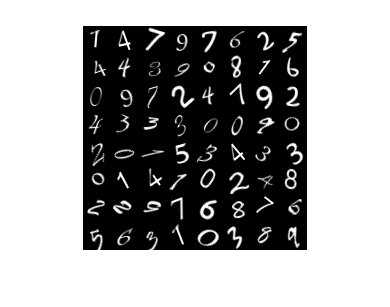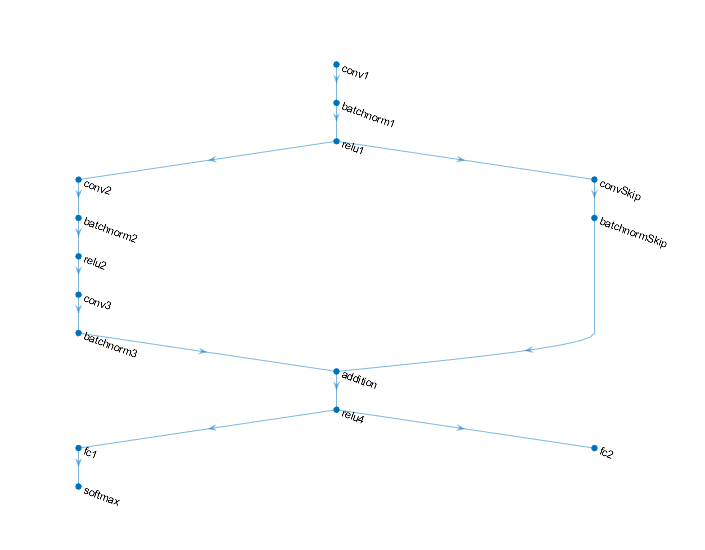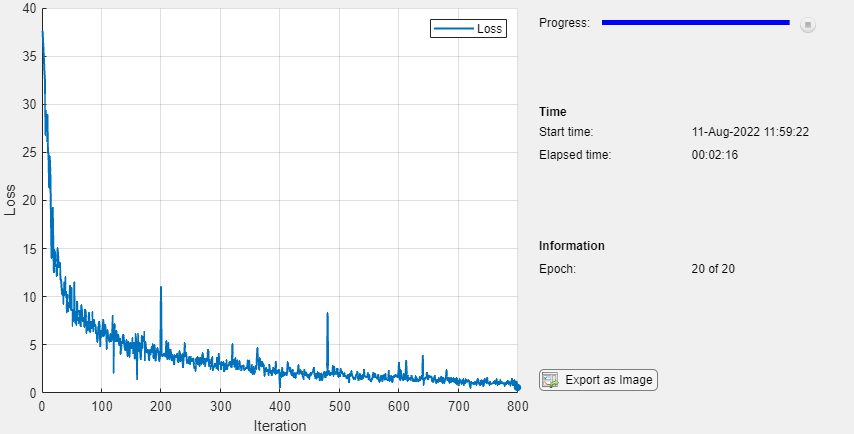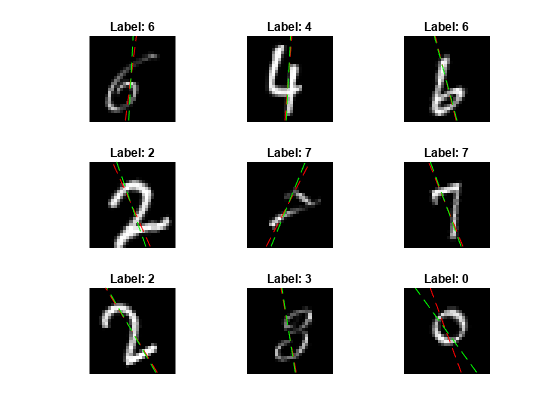# Train Network Using Model Function

This example shows how to create and train a deep learning network by using functions rather than a layer graph or a `dlnetwork`. The advantage of using functions is the flexibility to describe a wide variety of networks. The disadvantage is that you must complete more steps and prepare your data carefully. This example uses images of handwritten digits, with the dual objectives of classifying the digits and determining the angle of each digit from the vertical.

The `digitTrain4DArrayData` function loads the images, their digit labels, and their angles of rotation from the vertical. Create `arrayDatastore` objects for the images, labels, and angles, and then use the `combine` function to make a single datastore that contains all of the training data. Extract the class names and number of nondiscrete responses.

```[XTrain,YTrain,anglesTrain] = digitTrain4DArrayData; dsXTrain = arrayDatastore(XTrain,'IterationDimension',4); dsYTrain = arrayDatastore(YTrain); dsAnglesTrain = arrayDatastore(anglesTrain); dsTrain = combine(dsXTrain,dsYTrain,dsAnglesTrain); classNames = categories(YTrain); numClasses = numel(classNames); numResponses = size(anglesTrain,2); numObservations = numel(YTrain);```

View some images from the training data.

```idx = randperm(numObservations,64); I = imtile(XTrain(:,:,:,idx)); figure imshow(I)```### Define Deep Learning Model

Define the following network that predicts both labels and angles of rotation.

• A convolution-batchnorm-ReLU block with 16 5-by-5 filters.

• A branch of two convolution-batchnorm blocks each with 32 3-by-3 filters with a ReLU operation between

• A skip connection with a convolution-batchnorm block with 32 1-by-1 convolutions.

• Combine both branches using addition followed by a ReLU operation

• For the regression output, a branch with a fully connected operation of size 1 (the number of responses).

• For classification output, a branch with a fully connected operation of size 10 (the number of classes) and a softmax operation.#### Define and Initialize Model Parameters and State

Define the parameters for each of the operations and include them in a struct. Use the format `parameters.OperationName.ParameterName` where `parameters` is the struct, O`perationName` is the name of the operation (for example "conv1") and `ParameterName` is the name of the parameter (for example, "Weights").

Create a struct `parameters` containing the model parameters. Initialize the learnable layer weights and biases using the `initializeGlorot` and `initializeZeros` example functions, respectively. Initialize the batch normalization offset and scale parameters with the `initializeZeros` and `initializeOnes` example functions, respectively.

To perform training and inference using batch normalization layers, you must also manage the network state. Before prediction, you must specify the dataset mean and variance derived from the training data. Create a struct `state` containing the state parameters. The batch normalization statistics must not be `dlarray` objects. Initialize the batch normalization trained mean and trained variance states using the `zeros` and `ones` functions, respectively.

The initialization example functions are attached to this example as supporting files.

Initialize the parameters for the first convolutional layer.

```filterSize = [5 5]; numChannels = 1; numFilters = 16; sz = [filterSize numChannels numFilters]; numOut = prod(filterSize) * numFilters; numIn = prod(filterSize) * numFilters; parameters.conv1.Weights = initializeGlorot(sz,numOut,numIn); parameters.conv1.Bias = initializeZeros([numFilters 1]);```

Initialize the parameters and state for the first batch normalization layer.

```parameters.batchnorm1.Offset = initializeZeros([numFilters 1]); parameters.batchnorm1.Scale = initializeOnes([numFilters 1]); state.batchnorm1.TrainedMean = zeros(numFilters,1,'single'); state.batchnorm1.TrainedVariance = ones(numFilters,1,'single');```

Initialize the parameters for the second convolutional layer.

```filterSize = [3 3]; numChannels = 16; numFilters = 32; sz = [filterSize numChannels numFilters]; numOut = prod(filterSize) * numFilters; numIn = prod(filterSize) * numFilters; parameters.conv2.Weights = initializeGlorot(sz,numOut,numIn); parameters.conv2.Bias = initializeZeros([numFilters 1]);```

Initialize the parameters and state for the second batch normalization layer.

```parameters.batchnorm2.Offset = initializeZeros([numFilters 1]); parameters.batchnorm2.Scale = initializeOnes([numFilters 1]); state.batchnorm2.TrainedMean = zeros(numFilters,1,'single'); state.batchnorm2.TrainedVariance = ones(numFilters,1,'single');```

Initialize the parameters for the third convolutional layer.

```filterSize = [3 3]; numChannels = 32; numFilters = 32; sz = [filterSize numChannels numFilters]; numOut = prod(filterSize) * numFilters; numIn = prod(filterSize) * numFilters; parameters.conv3.Weights = initializeGlorot(sz,numOut,numIn); parameters.conv3.Bias = initializeZeros([numFilters 1]);```

Initialize the parameters and state for the third batch normalization layer.

```parameters.batchnorm3.Offset = initializeZeros([numFilters 1]); parameters.batchnorm3.Scale = initializeOnes([numFilters 1]); state.batchnorm3.TrainedMean = zeros(numFilters,1,'single'); state.batchnorm3.TrainedVariance = ones(numFilters,1,'single');```

Initialize the parameters for the convolutional layer in the skip connection.

```filterSize = [1 1]; numChannels = 16; numFilters = 32; sz = [filterSize numChannels numFilters]; numOut = prod(filterSize) * numFilters; numIn = prod(filterSize) * numFilters; parameters.convSkip.Weights = initializeGlorot(sz,numOut,numIn); parameters.convSkip.Bias = initializeZeros([numFilters 1]);```

Initialize the parameters and state for the batch normalization layer in the skip connection.

```parameters.batchnormSkip.Offset = initializeZeros([numFilters 1]); parameters.batchnormSkip.Scale = initializeOnes([numFilters 1]); state.batchnormSkip.TrainedMean = zeros([numFilters 1],'single'); state.batchnormSkip.TrainedVariance = ones([numFilters 1],'single');```

Initialize the parameters for the fully connected layer corresponding to the classificaiton output.

```sz = [numClasses 6272]; numOut = numClasses; numIn = 6272; parameters.fc1.Weights = initializeGlorot(sz,numOut,numIn); parameters.fc1.Bias = initializeZeros([numClasses 1]);```

Initialize the parameters for the fully connected layer corresponding to the regression output.

```sz = [numResponses 6272]; numOut = numResponses; numIn = 6272; parameters.fc2.Weights = initializeGlorot(sz,numOut,numIn); parameters.fc2.Bias = initializeZeros([numResponses 1]);```

View the struct of the parameters.

`parameters`
```parameters = struct with fields: conv1: [1×1 struct] batchnorm1: [1×1 struct] conv2: [1×1 struct] batchnorm2: [1×1 struct] conv3: [1×1 struct] batchnorm3: [1×1 struct] convSkip: [1×1 struct] batchnormSkip: [1×1 struct] fc1: [1×1 struct] fc2: [1×1 struct] ```

View the parameters for the "conv1" operation.

`parameters.conv1`
```ans = struct with fields: Weights: [5×5×1×16 dlarray] Bias: [16×1 dlarray] ```

View the struct of the state.

`state`
```state = struct with fields: batchnorm1: [1×1 struct] batchnorm2: [1×1 struct] batchnorm3: [1×1 struct] batchnormSkip: [1×1 struct] ```

View the state parameters for the "batchnorm1" operation.

`state.batchnorm1`
```ans = struct with fields: TrainedMean: [16×1 single] TrainedVariance: [16×1 single] ```

#### Define Model Function

Create the function `model`, listed at the end of the example, that computes the outputs of the deep learning model described earlier.

The function `model` takes the model parameters `parameters`, the input data `dlX`, the flag `doTraining` which specifies whether to model should return outputs for training or prediction, and the network state `state`. The network outputs the predictions for the labels, the predictions for the angles, and the updated network state.

Create the function `modelGradients`, listed at the end of the example, that takes the model parameters, a mini-batch of input data `dlX` with corresponding targets `T1` and `T2` containing the labels and angles, respectively, and returns the gradients of the loss with respect to the learnable parameters, the updated network state, and the corresponding loss.

### Specify Training Options

Specify the training options. Train for 20 epochs with a mini-batch size of 128.

```numEpochs = 20; miniBatchSize = 128;```

To monitor the training progress, you can plot the training loss after each iteration. Create the variable plots that contains "training-progress". If you do not want to plot the training progress, then set this value to "none".

`plots = "training-progress";`

### Train Model

Use `minibatchqueue` to process and manage the mini-batches of images. For each mini-batch:

• Use the custom mini-batch preprocessing function `preprocessMiniBatch` (defined at the end of this example) to one-hot encode the class labels.

• Format the image data with the dimension labels `'SSCB'` (spatial, spatial, channel, batch). By default, the `minibatchqueue` object converts the data to `dlarray` objects with underlying type `single`. Do not add a format to the class labels or angles.

• Train on a GPU if one is available. By default, the `minibatchqueue` onbject converts each output to a `gpuArray` if a GPU is available. Using a GPU requires Parallel Computing Toolbox™ and a CUDA® enabled NVIDIA® GPU with compute capability 3.0 or higher.

```mbq = minibatchqueue(dsTrain,... 'MiniBatchSize',miniBatchSize,... 'MiniBatchFcn', @preprocessMiniBatch,... 'MiniBatchFormat',{'SSCB','',''});```

For each epoch, shuffle the data and loop over mini-batches of data. At the end of each iteration, display the training progress. For each mini-batch:

• Evaluate the model gradients and loss using `dlfeval` and the `modelGradients` function.

• Update the network parameters using the `adamupdate` function.

```trailingAvg = []; trailingAvgSq = [];```

Initialize the training progress plot.

```if plots == "training-progress" figure lineLossTrain = animatedline('Color',[0.85 0.325 0.098]); ylim([0 inf]) xlabel("Iteration") ylabel("Loss") grid on end```

Train the model.

```iteration = 0; start = tic; % Loop over epochs. for epoch = 1:numEpochs % Shuffle data. shuffle(mbq) % Loop over mini-batches while hasdata(mbq) iteration = iteration + 1; [dlX,dlY1,dlY2] = next(mbq); % Evaluate the model gradients, state, and loss using dlfeval and the % modelGradients function. [gradients,state,loss] = dlfeval(@modelGradients, parameters, dlX, dlY1, dlY2, state); % Update the network parameters using the Adam optimizer. [parameters,trailingAvg,trailingAvgSq] = adamupdate(parameters,gradients, ... trailingAvg,trailingAvgSq,iteration); % Display the training progress. if plots == "training-progress" D = duration(0,0,toc(start),'Format','hh:mm:ss'); addpoints(lineLossTrain,iteration,double(gather(extractdata(loss)))) title("Epoch: " + epoch + ", Elapsed: " + string(D)) drawnow end end end```### Test Model

Test the classification accuracy of the model by comparing the predictions on a test set with the true labels and angles. Manage the test data set using a `minibatchqueue` object with the same setting as the training data.

```[XTest,YTest,anglesTest] = digitTest4DArrayData; dsXTest = arrayDatastore(XTest,'IterationDimension',4); dsYTest = arrayDatastore(YTest); dsAnglesTest = arrayDatastore(anglesTest); dsTest = combine(dsXTest,dsYTest,dsAnglesTest); mbqTest = minibatchqueue(dsTest,... 'MiniBatchSize',miniBatchSize,... 'MiniBatchFcn', @preprocessMiniBatch,... 'MiniBatchFormat',{'SSCB','',''});```

To predict the labels and angles of the validation data, loop over the mini-batches and use the model function with the `doTraining` option set to `false`. Store the predicted classes and angles. Compare the predicted and true classes and angles and store the results.

```doTraining = false; classesPredictions = []; anglesPredictions = []; classCorr = []; angleDiff = []; % Loop over mini-batches. while hasdata(mbqTest) % Read mini-batch of data. [dlXTest,dlY1Test,dlY2Test] = next(mbqTest); % Make predictions using the predict function. [dlY1Pred,dlY2Pred] = model(parameters,dlXTest,doTraining,state); % Determine predicted classes. Y1PredBatch = onehotdecode(dlY1Pred,classNames,1); classesPredictions = [classesPredictions Y1PredBatch]; % Dermine predicted angles Y2PredBatch = extractdata(dlY2Pred); anglesPredictions = [anglesPredictions Y2PredBatch]; % Compare predicted and true classes Y1Test = onehotdecode(dlY1Test,classNames,1); classCorr = [classCorr Y1PredBatch == Y1Test]; % Compare predicted and true angles angleDiffBatch = Y2PredBatch - dlY2Test; angleDiff = [angleDiff extractdata(gather(angleDiffBatch))]; end```

Evaluate the classification accuracy.

`accuracy = mean(classCorr)`
```accuracy = 0.9730 ```

Evaluate the regression accuracy.

`angleRMSE = sqrt(mean(angleDiff.^2))`
```angleRMSE = single 6.6909 ```

View some of the images with their predictions. Display the predicted angles in red and the correct labels in green.

```idx = randperm(size(XTest,4),9); figure for i = 1:9 subplot(3,3,i) I = XTest(:,:,:,idx(i)); imshow(I) hold on sz = size(I,1); offset = sz/2; thetaPred = anglesPredictions(idx(i)); plot(offset*[1-tand(thetaPred) 1+tand(thetaPred)],[sz 0],'r--') thetaValidation = anglesTest(idx(i)); plot(offset*[1-tand(thetaValidation) 1+tand(thetaValidation)],[sz 0],'g--') hold off label = string(classesPredictions(idx(i))); title("Label: " + label) end```### Model Function

The function `model` takes the model parameters `parameters`, the input data `dlX`, the flag `doTraining` which specifies whether to model should return outputs for training or prediction, and the network state `state`. The network outputs the predictions for the labels, the predictions for the angles, and the updated network state.

```function [dlY1,dlY2,state] = model(parameters,dlX,doTraining,state) % Convolution weights = parameters.conv1.Weights; bias = parameters.conv1.Bias; dlY = dlconv(dlX,weights,bias,'Padding','same'); % Batch normalization, ReLU offset = parameters.batchnorm1.Offset; scale = parameters.batchnorm1.Scale; trainedMean = state.batchnorm1.TrainedMean; trainedVariance = state.batchnorm1.TrainedVariance; if doTraining [dlY,trainedMean,trainedVariance] = batchnorm(dlY,offset,scale,trainedMean,trainedVariance); % Update state state.batchnorm1.TrainedMean = trainedMean; state.batchnorm1.TrainedVariance = trainedVariance; else dlY = batchnorm(dlY,offset,scale,trainedMean,trainedVariance); end dlY = relu(dlY); % Convolution, batch normalization (Skip connection) weights = parameters.convSkip.Weights; bias = parameters.convSkip.Bias; dlYSkip = dlconv(dlY,weights,bias,'Stride',2); offset = parameters.batchnormSkip.Offset; scale = parameters.batchnormSkip.Scale; trainedMean = state.batchnormSkip.TrainedMean; trainedVariance = state.batchnormSkip.TrainedVariance; if doTraining [dlYSkip,trainedMean,trainedVariance] = batchnorm(dlYSkip,offset,scale,trainedMean,trainedVariance); % Update state state.batchnormSkip.TrainedMean = trainedMean; state.batchnormSkip.TrainedVariance = trainedVariance; else dlYSkip = batchnorm(dlYSkip,offset,scale,trainedMean,trainedVariance); end % Convolution weights = parameters.conv2.Weights; bias = parameters.conv2.Bias; dlY = dlconv(dlY,weights,bias,'Padding','same','Stride',2); % Batch normalization, ReLU offset = parameters.batchnorm2.Offset; scale = parameters.batchnorm2.Scale; trainedMean = state.batchnorm2.TrainedMean; trainedVariance = state.batchnorm2.TrainedVariance; if doTraining [dlY,trainedMean,trainedVariance] = batchnorm(dlY,offset,scale,trainedMean,trainedVariance); % Update state state.batchnorm2.TrainedMean = trainedMean; state.batchnorm2.TrainedVariance = trainedVariance; else dlY = batchnorm(dlY,offset,scale,trainedMean,trainedVariance); end dlY = relu(dlY); % Convolution weights = parameters.conv3.Weights; bias = parameters.conv3.Bias; dlY = dlconv(dlY,weights,bias,'Padding','same'); % Batch normalization offset = parameters.batchnorm3.Offset; scale = parameters.batchnorm3.Scale; trainedMean = state.batchnorm3.TrainedMean; trainedVariance = state.batchnorm3.TrainedVariance; if doTraining [dlY,trainedMean,trainedVariance] = batchnorm(dlY,offset,scale,trainedMean,trainedVariance); % Update state state.batchnorm3.TrainedMean = trainedMean; state.batchnorm3.TrainedVariance = trainedVariance; else dlY = batchnorm(dlY,offset,scale,trainedMean,trainedVariance); end % Addition, ReLU dlY = dlYSkip + dlY; dlY = relu(dlY); % Fully connect, softmax (labels) weights = parameters.fc1.Weights; bias = parameters.fc1.Bias; dlY1 = fullyconnect(dlY,weights,bias); dlY1 = softmax(dlY1); % Fully connect (angles) weights = parameters.fc2.Weights; bias = parameters.fc2.Bias; dlY2 = fullyconnect(dlY,weights,bias); end```

The `modelGradients` function, takes the model parameters, a mini-batch of input data `dlX` with corresponding targets `T1` and `T2` containing the labels and angles, respectively, and returns the gradients of the loss with respect to the learnable parameters, the updated network state, and the corresponding loss.

```function [gradients,state,loss] = modelGradients(parameters,dlX,T1,T2,state) doTraining = true; [dlY1,dlY2,state] = model(parameters,dlX,doTraining,state); lossLabels = crossentropy(dlY1,T1); lossAngles = mse(dlY2,T2); loss = lossLabels + 0.1*lossAngles; gradients = dlgradient(loss,parameters); end```

### Mini-Batch Preprocessing Function

The `preprocessMiniBatch` function preprocesses the data using the following steps:

1. Extract the image data from the incoming cell array and concatenate into a numeric array. Concatenating the image data over the fourth dimension adds a third dimension to each image, to be used as a singleton channel dimension.

2. Extract the label and angle data from the incoming cell arrays and concatenate along the second dimension into a categorical array and a numeric array, respectively.

3. One-hot encode the categorical labels into numeric arrays. Encoding into the first dimension produces an encoded array that matches the shape of the network output.

```function [X,Y,angle] = preprocessMiniBatch(XCell,YCell,angleCell) % Extract image data from cell and concatenate X = cat(4,XCell{:}); % Extract label data from cell and concatenate Y = cat(2,YCell{:}); % Extract angle data from cell and concatenate angle = cat(2,angleCell{:}); % One-hot encode labels Y = onehotencode(Y,1); end```